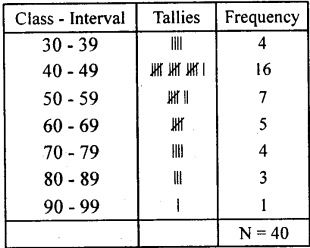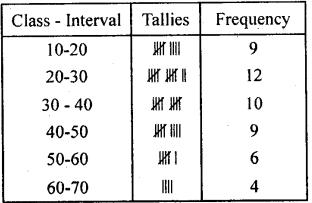# KSEEB Solutions for Class 8 Maths Chapter 13 Statistics Ex 13.1

In this chapter, we provide KSEEB SSLC Class 8 Maths Chapter 13 Statistics Ex 13.1 for English medium students, Which will very helpful for every student in their exams. Students can download the latest KSEEB SSLC Class 8 Maths Chapter 13 Statistics Ex 13.1 pdf, free KSEEB SSLC Class 8 Maths Chapter 13 Statistics Ex 13.1 pdf download. Now you will get step by step solution to each question.

### Karnataka State Syllabus Class 8 Maths Chapter 13 Statistics Ex 13.1

Question 1.
The marks scored by 40 candidates in an examination (out of 100) is given below.
75, 65, 57, 50, 32, 54, 75, 67, 75, 88, 80, 42, 40, 41, 34, 78, 43, 61, 42, 46, 68, 52, 43, 49, 59, 49, 67, 34, 33, 87, 97, 47, 46, 54, 48, 45, 51, 47, 41, 43.
Prepare a frequency distribution table with the class size 10. Take the class intervals as 30 – 39, 40 – 49 and answer the following questions.
(i) Which class intervals have highest and lowest frequency.
(ii) Write the upper and lower limits of the class interval 30 – 39.
(iii) What is the range of the given distribution?(i) The class interval 40-49 has highest frequency and 90-99 has the lowest frequency.
(ii) The lower limit of the class interval 30 – 39 is 29.5 and upper limit is 39.5
(iii) Range = Highest score – lowest score = 97- 32 = 65
Range = 65.

Question 2.
Prepare the frequency distribution table for the given set of scores. 39, 16, 30, 37, 53, 15, 16, 60, 58, 26, 28, 19, 20, 12, 14, 24, 59, 21, 57, 38, 25, 36,34, 15, 25, 41, 52, 45, 60, 63, 18, 26, 43, 18, 27, 59, 63, 46, 48, 25, 33, 46, 27, 46, 42, 48, 35, 64, 24 Take class intervals as (10 – 20), (20, 30)…. and answer the following.
(i) What does the frequency corresponding to the third class interval mean?
(ii) What is the size of each class interval? Find the midpoint of the class interval 30 – 40.
(iii) What is the range of the given set of scores?i) The frequency of third class interval 30 – 40 is 10
(ii) Size of each class interval is 10 and mid point of the class interval 30 – 40 is$\frac{(30+40)}{2}$ =$\frac { 70 }{ 2 }$ = 35
(iii) Range = 64 – 12 = 52.

All Chapter KSEEB Solutions For Class 8 maths

—————————————————————————–

All Subject KSEEB Solutions For Class 8

*************************************************

I think you got complete solutions for this chapter. If You have any queries regarding this chapter, please comment on the below section our subject teacher will answer you. We tried our best to give complete solutions so you got good marks in your exam.

If these solutions have helped you, you can also share kseebsolutionsfor.com to your friends.

Best of Luck!!